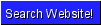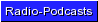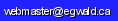Egwald Economics: Microeconomics

Oligopoly / Government Firm | Differentiated Oligopoly | Monopolistic Competition

Imperfect Competition Models

Monopolistic Competition

by

Elmer G. Wiens

Egwald's popular web pages are provided without cost to users.
Please show your support by joining Egwald Web Services as a Facebook Fan:Follow Elmer Wiens on Twitter:The many firms in a monopolistically competitive industry produce differentiated yet similar products. New firms can easily enter the industry. Textbooks often give retail trade or the hotel industry as examples.

A monopolistically competitive firm's own demand curve is highly elastic, permitting it to vary its price within a narrow range of prices. The other firms' products are either very close substitutes or, a large number of other firms' products are substitutes (not necessarily very close substitutes).

The diagram below shows the industry and firm's demand and marginal revenue curves. The slopes of the firm's demand and marginal revenue curves are greatly exaggerated. In reality, they are almost coincident horizontal lines. The firm's own demand schedule intersects the "industry's (average) demand schedule" at the firm's "equilibrium level of output and price," given the output levels of the other firms in the industry. These concepts are explained below.

Monopolistic Competition

Industry and Firm Demand CurvesAlmost every day I get "flyers" in the mail informing me about the products of a new or existing restaurant, clothing store, or laundry, and their terms of trade (relative prices). Monopolistically competitive firms advertise (unlike perfectly competitive firms). Advertising seems necessary for a firm to enter and to remain in the industry.

Retail firms pay taxes on the value of products sold (in Canada - G.S.T. and provincial sales taxes). The effect of varying these taxes on firms will be built into the model of a monopolistic industry.

Suppose there are n firms in the industry. If q is the output of product and v is the amount of advertising of a typical firm, then the price, p, of its product is:

p(q,v) = A / (n * q + B) + ad(v)

A and B are positive constants. Since we are modelling the typical firm, in equilibrium each of the n firms will sell the same amount of product. Each firm's advertising function ad(v) shifts the demand curve. It is given by:

where c1 and c2 are positive constants. The negative sign ensures diminishing returns to advertising. Without advertising, a firm's sales prospects are diminished.

Including the term, B, as a positive constant in the "industry's (average) demand schedule" ensures a "finite price," even when n = 0, or q = 0.

The Monopolistic Competition model on this page is in some ways similar to the model of monopolistic competition of Henderson and Quandt (235-239). It uses a functional form for the demand functions of the firms that can be found in R.G.D. Allen (114).

The cost function for the typical firm is:

c(q,v) = a*q2 + b*q + c + s*v

where a, b, c, and s are positive constants. Fixed costs are (c + s*v) because they do not vary with q.

The average cost function will be U-shaped:

ac(q,v) = a*q + b + (c + s*v) / q

With an ad valorem tax at rate t (sales tax rate = t / (1-t)), the revenue to the firm is:

r(q,v) = q * p(q,v) * (1 - t)

At given levels of output, q, and advertising, v, the after tax profit of the typical firm is given by:

prof(q,v) = r(q,v) - c(q,v)

If the firm sets q and v so as to maximize its profit, the following first order marginal conditions must obtain:

profq = rq - cq = 0

profv = rv - cv = 0

where the subscripts represent the partial derivative of the function with respect to the specified variable.

These first order conditions plus their associated Jacobian are used for each set of parameters of the model to solve for the optimal value of q and v.

Suppose, first, that all firms in the industry behave (in concert) as the typical firm. Each firm will attempt to satisfy the above first order conditions by selecting appropriate levels of q and v.

Firms will enter (or exit) the industry until the after tax price equals the average cost at the equilibrium level of q, the typical firm's output. At this equilibrium q and equilibrium number of firms n, the average cost curve will be tangent to the average revenue curve, p(q,v), and profits ~= 0.

Such an equilibrium is shown below.

Monopolistic Competition - 37 Firms
Firm Equilibrium
demand for product after tax
p = (3000 / ( 37 * 11.41 + 50) + 7.43) * (1 - 0.07)
cost
cost = 0.26 q2 + 1.6 q + 70 + 5 v
ad = 2.6 v - 0.22 v2
before tax p = 13.78,  after tax p = 12.82
q = 11.41,   tax rate = 0.07
v = 4.84,   ad = 7.43,   s = 5
after tax revenue = 146.27
cost = 146.3
profit = -0.04However, the typical firm's own demand schedule is given by:

p(q,x,v) = A / ((n-1) * q + x + B) + ad(v)

where the other (n-1) firms are at their equilibrium level of output q, while the typical firm varies its own output x.

In the diagram above, we can see that the firm's own average revenue curve, p(q,x,v), intersects the ac(q,v) and p(q,v) curves at their point of tangency. Here the firm's own marginal revenue, mr(q,x,v,) curve lies above its marginal cost curve, mc(x,v). Thus, it may try to increase its sales by cutting its price slightly. If the other firms follow suit, this price cutting will lead to an actual loss in revenue and an increase in the firm's costs with profit turning negative. Some firms might exit, or firms might increase prices to their former level, restoring the zero profit condition.

If the industry's firms are aggressive enough, we might expect, provided it is feasible, another "equilibrium" to obtain: with sales at that level of ouput where each firm's own average revenue curve, p(q,x,v), is tangent to its own average cost curve, ac(x,v), and where its own marginal revenue, mr(q,x,v), equals marginal cost, mc(x,v).

This is the equilibrium below. You can adjust the values of the parameters of the model with the form below if you so desire to see how the equilbium changes.

Monopolistic Competition - 30 Firms
Firm Equilibrium
demand for product after tax
p = (3000 / ( 30 * 18.92 + 50) + 7.59) * (1 - 0.07)
cost
cost = 0.26 q2 + 1.6 q + 70 + 5 v
ad = 2.6 v - 0.22 v2
before tax p = 12.45,  after tax p = 11.58
q = 18.92,   tax rate = 0.07
v = 5.26,   ad = 7.59,   s = 5
after tax revenue = 219.02
cost = 219.65
profit = -0.63The industry's price as a function of n, q, and v is: p(q,v) = A / (n * q + B) + ad(v),         where advertising as a function of v is: ad(v) = c1*v - c2*v2 A firm's costs as a function of q and v is: c(q,v) = a*q2 + b*q + c + s*v. A firm's revenue as a function of q and v is: r(q,v) = q * p(q,v) * (1 - t). A firm's own demand schedule as a function of x and v is: p(q,x,v) = A / ((n-1) * q + x + B) + ad(v). A firm's own costs as a function of x and v is: c(x,v) = a*x2 + b*x + c + s*v. A firm's own revenue as a function of x and v is: r(q,x,v) = x * p(q,x,v) * (1 - t).
 Adjust Coefficients Reset value of n [3, 50] 30 Reset value of A [2500, 4000] 3000 Reset value of b [ .8, 3] 1.6 Reset value of c [40, 90] 70 Reset value of t [0, .15] 0.07 Reset value of s [1, 6] 5
 Try adjusting the fixed cost parameter, c, to achieve profit = 0. With lower fixed costs, c, what adjustments might be necessary to the coefficients, a and b, of a firm's cost function? Why? Will a monopolistically competitive firm, in "equilibrium," produce output at the minimum of its short run average cost curve? See what happens when you adjust s, the parameter of the cost function measuring the cost of advertising. Does it make sense to talk about the long run average cost schedule of a monopolistically competitive firm? What happens when you set the number of firms n = 4? Is this a good model for an oligopoly?

Solving the Model

The first order conditions for profit maximization consist of the following two nonlinear equations:

L1 = profq = rq(q,v) - cq(q,v) = 0

L2 = profv = rv(q,v) - cv(q,v) = 0

where

rq(q,v) = [p(q,v) + q * pq(q,v)] * [1 - t]

rv(q,v) = [q * pv(q,v)] * [1 -t]

cq(q,v) = 2*a*q + b

cv(q,v) = s

pq(q,v) = - n*A / (n*q + B)2, or

px(q,x,v) = -A / ((n-1)*q + x + B)2

Note: in equilibrium, q = x.

The Jacobian matrix of these equations is (differentiating wrt x when appropriate):

 || L1q L1v || || L2q L2v ||

The gradient and Newton methods use the first order equations and the Jacobian matrix to solve for the equilibrium - profit maximizing - values of q and v.

Works Cited and Consulted

• Allen, R.G.D. Mathematical Analysis for Economists. London: MacMillan, 1938.

• Archibald, G.C. The Theory of the Firm. Middlesex: Penguin, 1971

• Bain, Joe S. Price Theory. New York: Wiley, 1952

• Henderson, James M. and Richard E. Quandt. Microeconomic Theory: A Mathematical Approach. New York: McGraw-Hill, 1971.

• Hirshleifer, Jack. Price Theory and Applications. Englewood Cliffs: Prentice-Hall, 1976.Copyright © Elmer G. Wiens:   Egwald Web ServicesAll Rights Reserved.    Inquiries